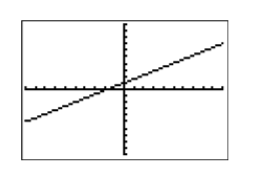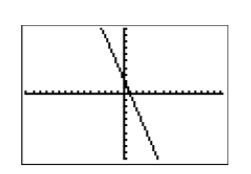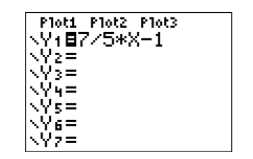# 7.3: Slope-Intercept Form of a Line

$$\newcommand{\vecs}{\overset { \rightharpoonup} {\mathbf{#1}} }$$ $$\newcommand{\vecd}{\overset{-\!-\!\rightharpoonup}{\vphantom{a}\smash {#1}}}$$$$\newcommand{\id}{\mathrm{id}}$$ $$\newcommand{\Span}{\mathrm{span}}$$ $$\newcommand{\kernel}{\mathrm{null}\,}$$ $$\newcommand{\range}{\mathrm{range}\,}$$ $$\newcommand{\RealPart}{\mathrm{Re}}$$ $$\newcommand{\ImaginaryPart}{\mathrm{Im}}$$ $$\newcommand{\Argument}{\mathrm{Arg}}$$ $$\newcommand{\norm}{\| #1 \|}$$ $$\newcommand{\inner}{\langle #1, #2 \rangle}$$ $$\newcommand{\Span}{\mathrm{span}}$$ $$\newcommand{\id}{\mathrm{id}}$$ $$\newcommand{\Span}{\mathrm{span}}$$ $$\newcommand{\kernel}{\mathrm{null}\,}$$ $$\newcommand{\range}{\mathrm{range}\,}$$ $$\newcommand{\RealPart}{\mathrm{Re}}$$ $$\newcommand{\ImaginaryPart}{\mathrm{Im}}$$ $$\newcommand{\Argument}{\mathrm{Arg}}$$ $$\newcommand{\norm}{\| #1 \|}$$ $$\newcommand{\inner}{\langle #1, #2 \rangle}$$ $$\newcommand{\Span}{\mathrm{span}}$$$$\newcommand{\AA}{\unicode[.8,0]{x212B}}$$

We start with the definition of the $$y$$-intercept of a line.

The $$y$$-intercept

The point $$(0,b)$$ where the graph of a line crosses the $$y$$-axis is called the $$y$$-intercept of the line.

We will now generate the slope-intercept formula for a line having $$y$$-intercept $$(0,b)$$ and $$\text {Slope} = m$$ (see Figure $$\PageIndex{1}$$). Let $$(x,y)$$ be an arbitrary point on the line.Figure $$\PageIndex{1}$$: Line with $$y$$-intercept at $$(0,b)$$ and $$\text {Slope} = m$$.

Start with the fact that the slope of the line is the rate at which the dependent variable is changing with respect to the independent variable.

$\text {Slope} =\dfrac{\Delta y}{\Delta x} \qquad \color{Red} \text {Slope formula.} \nonumber$

Substitute $$m$$ for the slope. To determine both the change in $$y$$ and the change in $$x$$, subtract the coordinates of the point $$(0,b)$$ from the point $$(x,y)$$.

\begin{aligned} m&=\frac{y-b}{x-0} \quad \color {Red} \text { Substitute } m \text { for the Slope. } \Delta y =y-b \quad \color {Red} \text { and } \Delta x=x-0 .\\ m&=\frac{y-b}{x} \quad \color {Red}\text { Simplify. } \end{aligned} \nonumber

Clear fractions from the equation by multiplying both sides by the common denominator.

\begin{aligned} mx &= \left[\dfrac{y-b}{x}\right] x \quad \color {Red} \text { Multiply both sides by } x \\ mx &= y-b \quad \color {Red} \text { Cancel. } \end{aligned} \nonumber

Solve for $$y$$.

\begin{aligned} mx+b&= y-b+b \quad \color {Red} \text { Add } b \text { to both sides. } \\ mx+b&= y \quad \color {Red} \text { Simplify. } \end{aligned} \nonumber

Thus, the equation of the line is $$y = mx + b$$.

The Slope-Intercept form of a line

The equation of the line having $$y$$ intercept $$(0,b)$$ and slope $$m$$ is: $y = mx + b \nonumber$Because this form of a line depends on knowing the slope $$m$$ and the intercept $$(0,b)$$, this form is called the slope-intercept form of a line.

Example $$\PageIndex{1}$$

Sketch the graph of the line having equation $$y=\dfrac{3}{5} x+1$$.

Solution

Compare the equation $$y=\dfrac{3}{5} x+1$$ with the slope-intercept form $$y = mx + b$$, and note that $$m =3 /5$$ and $$b = 1$$. This means that the slope is $$3/5$$ and the $$y$$-intercept is $$(0,1)$$. Start by plotting the $$y$$-intercept $$(0,1)$$, then move uward $$3$$ units and right $$5$$ units, arriving at the point $$(5,4)$$. Draw the line through the points $$(0,1)$$ and $$(5,4)$$, then label it with its equation $$y=\dfrac{3}{5} x+1$$.Figure $$\PageIndex{2}$$: Hand-drawn graph of $$y=\dfrac{3}{5} x+1$$.Figure $$\PageIndex{3}$$: Select 5:ZSquare from the ZOOM menu to draw the graph of $$y=\dfrac{3}{5} x+1$$.

When we compare the calculator image in Figure $$\PageIndex{3}$$ with the hand-drawn graph in Figure $$\PageIndex{2}$$, we get a better match.

Exercise $$\PageIndex{1}$$

Sketch the graph of the line having equation $$y=-\dfrac{4}{3} x-2$$.Example $$\PageIndex{2}$$

Sketch the line with $$y$$-intercept $$(0,2)$$ and slope $$−7/3$$. Label the line with the slope-intercept form of its equation.

Solution

Plot the $$y$$-intercept $$(0,2)$$. Now use the slope $$−7/3$$. Start at $$(0,2)$$, then move down seven units, followed by a three unit move to the right to the point $$(3,−5)$$. Draw the line through the points $$(0,2)$$ and $$(3,−5)$$. (See Figure $$\PageIndex{4}$$).

Next, the $$y$$-intercept is $$(0,2)$$, so $$b = 2$$. Further, the slope is $$−7/3$$, so $$m = −7/3$$. Substitute these numbers into the slope-intercept form of the line.

\begin{aligned} y&= mx+b \quad \color {Red} \text { Slope-intercept form. } \\ y&= -\dfrac{7}{3} x+2 \quad \color {Red} \text { Substitute: }-7 / 3 \text { for } m, 2 \text { for } b . \end{aligned} \nonumber

Therefore, the slope-intercept form of the line is $$y=-\dfrac{7}{3} x+2$$. Label the line with this equation.Figure $$\PageIndex{4}$$: Hand-drawn graph of $$y=-\dfrac{7}{3} x+2$$

Check: To graph $$y=-\dfrac{7}{3} x+2$$, enter $$-7 / 3 * X+2$$ in Y1 in the Y= menu. Select 6:ZStandard from the ZOOM menu, followed by 5:ZSquare from the ZOOM menu to produce the graph shown in Figure $$\PageIndex{6}$$.Figure $$\PageIndex{5}$$: Hand-drawn graph of $$y=-\dfrac{7}{3} x+2$$Figure $$\PageIndex{6}$$: Select 6:ZStandard from the ZOOM menu, followed by 5:ZSquare from the ZOOM menu to produce the graph of the equation $$y=-\dfrac{7}{3} x+2$$

This provides a good match of the hand-drawn graph in Figure $$\PageIndex{5}$$ and our graphing calculator result in Figure $$\PageIndex{6}$$.

Exercise $$\PageIndex{2}$$

Sketch the line with $$y$$-intercept $$(0,−3)$$ and slope $$5/2$$. Label the line with the slope-intercept form of its equation.Example $$\PageIndex{3}$$

Use the graph of the line in the following figure to find the equation of the line.Solution

Note that the $$y$$-intercept of the line is $$(0,−1)$$ (See Figure $$\PageIndex{7}$$). Next, we try to locate a point on the line that passes directly through a lattice point, a point where a vertical and horizontal grid line intersect. In Figure $$\PageIndex{7}$$, we chose the point $$(5,6)$$. Now, starting at the $$y$$-intercept $$(0,1)$$, we move up $$7$$ units, then to the right $$5$$ units. Hence, the slope is $$m=\Delta y / \Delta x$$, or $$m =7 /5$$.

Note

We could also subtract the coordinates of point $$(0,−1)$$ from the coordinates of point $$(5,6)$$ to determine the slope. $\dfrac{6-(-1)}{5-0}=\dfrac{7}{5} \nonumber$Figure $$\PageIndex{7}$$: The line has $$y$$-intercept $$(0,−1)$$ and slope $$7/5$$.

Next, state the slope-intercept form, the substitute $$7/5$$ for $$m$$ and $$−1$$ for $$b$$.

\begin{aligned} y&= mx+b \quad \color {Red} \text { Slope-intercept form. } \\ y&= \dfrac{7}{5} x+(-1) \quad \color {Red} \text { Substitute: } 7 / 5 \text { for } m,-1 \text { for } b \end{aligned} \nonumber

Thus, the equation of the line is $$y=\dfrac{7}{5} x-1$$.

Check: This is an excellent situation to run a check on your graphing calculator.Figure $$\PageIndex{8}$$: Enter $$y=\dfrac{7}{5} x-1$$ in the Y= menu.Figure $$\PageIndex{9}$$: Select 6:ZStandard followed by 5:ZSquare (both from the ZOOM menu) to produce this graph.

When we compare the result in Figure $$\PageIndex{9}$$ with the original hand-drawn graph (see Figure $$\PageIndex{7}$$), we’re confident we have a good match.

Exercise $$\PageIndex{3}$$

Use the graph of the line in the figure below to find the equation of the line.$$y=-\dfrac{3}{5} x+3$$

## Applications

Let’s look at a linear application.

Example $$\PageIndex{4}$$

Jason spots his brother Tim talking with friends at the library, located $$300$$ feet away. He begins walking towards his brother at a constant rate of $$2$$ feet per second ($$2$$ ft/s).

1. Express the distance $$d$$ between Jason and his brother Tim in terms of the time $$t$$.
2. At what time after Jason begins walking towards Tim are the brothers $$200$$ feet apart?

Solution

Because the distance between Jason and his brother is decreasing at a constant rate, the graph of the distance versus time is a line. Let’s begin by making a rough sketch of the line. In Figure $$\PageIndex{10}$$, note that we’ve labeled what are normally the $$x$$- and $$y$$-axes with the time $$t$$ and distance $$d$$, and we’ve included the units with our labels.Figure $$\PageIndex{10}$$: A plot of the distance $$d$$ separating the brothers versus time $$t$$.

Let $$t = 0$$ seconds be the time that Jason begins walking towards his brother Tim. At time $$t = 0$$, the initial distance between the brothers is $$300$$ feet. This puts the $$d$$-intercept (normally the $$y$$-intercept) at the point $$(0,300)$$ (see Figure $$\PageIndex{10}$$).

Because Jason is walking toward his brother, the distance between the brothers decreases at a constant rate of $$2$$ feet per second. This means the line must slant downhill, making the slope negative, so $$m = −2$$ ft/s. We can construct an accurate plot of distance versus time by starting at the point $$(0,300)$$, then descending $$\Delta d=-300$$, then moving to the right $$\Delta t=150$$. This makes the slope $$\Delta d / \Delta t=-300 / 150=-2$$ (See Figure $$\PageIndex{10}$$). Note that the slope is the rate at which the distance $$d$$ between the brothers is changing with respect to time $$t$$.

Finally, the equation of the line is $$y = mx + b$$, where $$m$$ is the slope of the line and $$b$$ is the $$y$$-coordinate (in this case the $$d$$-coordinate) of the point where the graph crosses the vertical axis. Thus, substitute $$−2$$ for $$m$$, and $$300$$ for $$b$$ in the slope-intercept form of the line.

\begin{aligned} y&= mx+b \quad \color {Red} \text { Slope-intercept form. } \\ y&= -2x+300 \quad \color {Red} \text { Substitute: }-2 \text { for } m, 300 \text { for } b \end{aligned} \nonumber

One problem remains. The equation $$y = −2x + 300$$ gives us $$y$$ in terms of $$x$$.

1. The question required that we express the distance $$d$$ in terms of the time $$t$$. So, to finish the solution, replace $$y$$ with $$d$$ and $$x$$ with $$t$$ (check the axes labels in Figure $$\PageIndex{10}$$) to obtain a solution: $d=-2t+300 \nonumber$
2. Now that our equation expresses the distance between the brothers in terms of time, let’s answer part (b), “At what time after Jason begins walking towards Tim are the brothers $$200$$ feet apart?” To find this time, substitute $$200$$ for $$d$$ in the equation $$d =−2t + 300$$, then solve for $$t$$.\begin{aligned} d &= -2t+300 \quad \color {Red} \text { Distance equation } \\ 200 &= -2t+300 \quad \color {Red} \text { Substitute } 200 \text { for } d \end{aligned} \nonumberSolve this last equation for the time $$t$$.\begin{aligned} 200-300&= -2t+300-300 \quad \color {Red} \text { Subtract } 300 \text { from both sides. } \\ -100&= -2t \quad \color {Red} \text { Simplify both sides. } \\ \dfrac{-100}{-2}&= \dfrac{-2 t}{-2} \quad \color {Red} \text { Divide both sides by }-2 \\ 50&= t \quad \color {Red} \text { Simplify both sides. } \end{aligned} \nonumberThus, it takes Jason $$50$$ seconds to close the distance between the brothers to $$200$$ feet.

Exercise $$\PageIndex{4}$$

A swimmer is $$50$$ feet from the beach, and then begins swimming away from the beach at a constant rate of $$1.5$$ feet per second ($$1.5$$ ft/s). Express the distance $$d$$ between the swimmer and the beach in terms of the time $$t$$.

$$d=1.5 t+50$$